# CLASS 11 MATHS CHAPTER-12 INTRODUCTION TO THREE DIMENSIONAL GEOMETRY

### In each of the following Exercises 1 to 4, find the equations :

Question1. A point is on the x-axis. What are its y-coordinates and z-coordinates?

Solution :
If a point is on the x-axis, then its y-coordinates and z-coordinates are zero.

Question2. A point is in the X Z-plane. What can you say about its y-coordinate?

Solution :
If a point is in the X Z plane, then its y-coordinate is zero.

Question3. Name the octants in which the following points lie:

(1, 2, 3), (4, –2, 3), (4, –2, –5), (4, 2, –5), (–4, 2, –5), (–4, 2, 5),  (–3, –1, 6), (2, –4, –7)

Solution :
Thex-coordinate, y-coordinate, and z-coordinate of point (1, 2, 3) are all positive. Therefore, this point lies in octant I.

Thex-coordinate, y-coordinate, and z-coordinate of point (4, –2, 3) are positive, negative, and positive respectively. Therefore, this point lies in octant IV.

Thex-coordinate, y-coordinate, and z-coordinate of point (4, –2, –5) are positive, negative, and negative respectively. Therefore, this point lies in octant VIII.

Thex-coordinate, y-coordinate, and z-coordinate of point (4, 2, –5) are positive, positive, and negative respectively. Therefore, this point lies in octant V.

Thex-coordinate, y-coordinate, and z-coordinate of point (–4, 2, –5) are negative, positive, and negative respectively. Therefore, this point lies in octant VI.

Thex-coordinate, y-coordinate, and z-coordinate of point (–4, 2, 5) are negative, positive, and positive respectively. Therefore, this point lies in octant II.

Thex-coordinate, y-coordinate, and z-coordinate of point (–3, –1, 6) are negative, negative, and positive respectively. Therefore, this point lies in octant III.

Thex-coordinate, y-coordinate, and z-coordinate of point (2, –4, –7) are positive, negative, and negative respectively. Therefore, this point lies in octant VIII.

Question4. Fill in the blanks:

(i) The x-axis and y-axis taken together determine a plane known as_______.
(ii) The coordinates of points in the XY-plane are of the form _______.
(iii) Coordinate planes divide the space into ______ octants.

Solution :
(i) The x-axis and y-axis taken together determine a plane known as XY-plane.

(ii) The coordinates of points in the XY-plane are of the form (x, y, 0).

(iii) Coordinate planes divide the space into eight octants.

### In each of the following Exercises 1 to 4, find the equations :

Question1. Find the distance between the following pairs of points:

(i) (2, 3, 5) and (4, 3, 1)

(ii) (–3, 7, 2) and (2, 4, –1)

(iii) (–1, 3, –4) and (1, –3, 4)

(iv) (2, –1, 3) and (–2, 1, 3)

Solution :

The distance between points P(x1y1z1) and P(x2y2z2) is given by
(i)  Distance between points (2, 3, 5) and (4, 3, 1)

(ii) Distance between points (–3, 7, 2) and (2, 4, –1)

(iii) Distance between points (–1, 3, –4) and (1, –3, 4)

(iv) Distance between points (2, –1, 3) and (–2, 1, 3)

Question2. Show that the points (–2, 3, 5), (1, 2, 3) and (7, 0, –1) are collinear.

Solution :
Let points (–2, 3, 5), (1, 2, 3), and (7, 0, –1) be denoted by P, Q, and R respectively.

Points P, Q, and R are collinear if they lie on a line.

Here, PQ + QR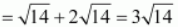= PR

Hence, points P(–2, 3, 5), Q(1, 2, 3), and R(7, 0, –1) are collinear.

Question3. Verify the following:

(i) (0, 7, –10), (1, 6, –6) and (4, 9, –6) are the vertices of an isosceles triangle.

(ii) (0, 7, 10), (–1, 6, 6) and (–4, 9, 6) are the vertices of a right angled triangle.

(iii) (–1, 2, 1), (1, –2, 5), (4, –7, 8) and (2, –3, 4) are the vertices of a parallelogram.

Solution :
(i) Let points (0, 7, –10), (1, 6, –6), and (4, 9, –6) be denoted by A, B, and C respectively.

Here, AB = BC ≠ CA

Thus, the given points are the vertices of an isosceles triangle.

(ii) Let (0, 7, 10), (–1, 6, 6), and (–4, 9, 6) be denoted by A, B, and C respectively.

Therefore, by Pythagoras theorem, ABC is a right triangle.

Hence, the given points are the vertices of a right-angled triangle.

(iii) Let (–1, 2, 1), (1, –2, 5), (4, –7, 8), and (2, –3, 4) be denoted by A, B, C, and D respectively.

Here, AB = CD = 6, BC = AD =43

Hence, the opposite sides of quadrilateral ABCD, whose vertices are taken in order, are equal.

Therefore, ABCD is a parallelogram.

Hence, the given points are the vertices of a parallelogram.

Question4. Find the equation of the set of points which are equidistant from the points (1, 2, 3) and (3, 2, –1).

Solution :
Let P (x,y, z) be the point that is equidistant from points A(1, 2, 3) and B(3, 2, –1).

Accordingly, PA = PB

⇒ x2 – 2x + 1 + y2 – 4+ 4 + z2 – 6z + 9 = x2 – 6x + 9 + y2 – 4y + 4 + z2 + 2z + 1

⇒ –2x –4y – 6z + 14 = –6x – 4y + 2+ 14

⇒ – 2x – 6z + 6x – 2z = 0

⇒ 4x – 8z = 0

⇒ x – 2z = 0

Thus, the required equation is x – 2z = 0.

Question5. Find the equation of the set of points P, the sum of whose distances from A (4, 0, 0) and B (–4, 0, 0) is equal to 10.

Solution :
Let the coordinates of P be (x,y,z).

The coordinates of points A and B are (4, 0, 0) and (–4, 0, 0) respectively.

It is given that PA + PB = 10.

On squaring both sides, we obtain

On squaring both sides again, we obtain

25 (x2 + 8x + 16 +y2 +z2) = 625 + 16x2 + 200x

⇒ 25x2 + 200x + 400 + 25y2 + 25z2 = 625 + 16x2 + 200x

⇒ 9x2 + 25y2 + 25z– 225 = 0

Thus, the required equation is 9x2 + 25y2 + 25z2 – 225 = 0.

### In each of the following Exercises 1 to 4, find the equations :

Question 1. Find the coordinates of the point which divides the line segment joining the points (–2, 3, 5) and (1, –4, 6) in the ratio

(i) 2:3 internally,

(ii) 2:3 externally.

Solution :
(i) The coordinates of point R that divides the line segment joining points P (x1,y1,z1) and Q (x2,y2,z2) internally in the ratiom:n are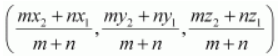.

Let R (x, y,z) be the point that divides the line segment joining points(–2, 3, 5) and (1, –4, 6) internally in the ratio 2:3

Thus, the coordinates of the required point are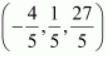.

(ii) The coordinates of point R that divides the line segment joining points P (x1,y1,z1) and Q (x2,y2,z2) externally in the ratiom:n are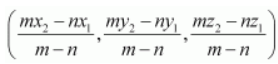.

Let R (x, y,z) be the point that divides the line segment joining points(–2, 3, 5) and (1, –4, 6) externally in the ratio 2:3

Thus, the coordinates of the required point are (–8, 17, 3).

Question2. Given that P (3, 2, –4), Q (5, 4, –6) and R (9, 8, –10) are collinear. Find the ratio in which Q divides PR.

Solution :
Let point Q (5, 4, –6) divide the line segment joining points P (3, 2, –4) and R (9, 8, –10) in the ratiok:1.

Therefore, by section formula,

Thus, point Q divides PR in the ratio 1:2.

Question 3. Find the ratio in which the Y Z-plane divides the line segment formed by joining the points (–2, 4, 7) and (3, –5, 8).

Solution :
Let the YZ planedivide the line segment joining points (–2, 4, 7) and (3, –5, 8) in the ratiok:1.

Hence, by section formula, the coordinates of point of intersection are given by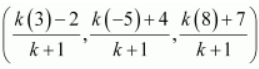On the Y Z plane, thex-coordinate of any point is zero.

Thus, the YZ plane divides the line segment formed by joining the given points in the ratio 2:3.

Question4. Using section formula, show that the points A (2, –3, 4), B (–1, 2, 1) and are collinear.

Solution :
The given points are A (2, –3, 4), B (–1, 2, 1), and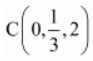.

Let P be a point that divides AB in the ratio k:1.

Hence, by section formula, the coordinates of P are given by

Now, we find the value ofk at which point P coincides with point C.

By taking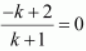, we obtaink = 2.

Fork = 2, the coordinates of point P are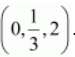.

i.e.,is a point that divides AB externally in the ratio 2:1 and is the same as point P.

Hence, points A, B, and C are collinear.

Question5. Find the coordinates of the points which trisect the line segment joining the points P (4, 2, –6) and Q (10, –16, 6).

Solution :
Let A and B be the points that trisect the line segment joining points P (4, 2, –6) and Q (10, –16, 6)

Point A divides PQ in the ratio 1:2. Therefore, by section formula, the coordinates of point A are given by

Point B divides PQ in the ratio 2:1. Therefore, by section formula, the coordinates of point B are given by

Thus, (6, –4, –2) and (8, –10, 2) are the points that trisect the line segment joining points P (4, 2, –6) and Q (10, –16, 6).

### Miscellaneous Exercise

Question1. Three vertices of a parallelogram ABCD are A (3, –1, 2), B (1, 2, –4) andC (–1, 1, 2). Find the coordinates of the fourth vertex.

Solution :
The three vertices of a parallelogram ABCD are given as A (3, –1, 2), B (1, 2, –4), and C (–1, 1, 2). Let the coordinates of the fourth vertex be D (x,y,z).

We know that the diagonals of a parallelogram bisect each other.

Therefore, in parallelogram ABCD, AC and BD bisect each other.

∴Mid-point of AC = Mid-point of BD

⇒x = 1,y = –2, andz = 8

Thus, the coordinates of the fourth vertex are (1, –2, 8).

Question2. Find the lengths of the medians of the triangle with vertices A (0, 0, 6), B (0, 4, 0) and (6, 0, 0).

Solution :
Let AD, BE, and CF be the medians of the given triangle ABC.

Since AD is the median, D is the mid-point of BC.

∴Coordinates of point D =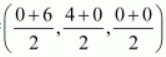= (3, 2, 0)

Thus, the lengths of the medians of ΔABC are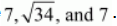.

Question3. If the origin is the centroid of the triangle PQR with vertices P (2a, 2, 6), Q (–4, 3b, –10) and R (8, 14, 2c), then find the values ofa,b andc.

Solution :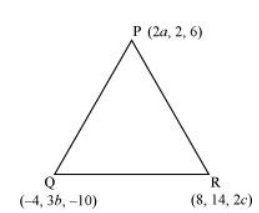It is known that the coordinates of the centroid of the triangle, whose vertices are (x1,y1,z1),  (x2,y2,z2) and (x3,y3,z3), are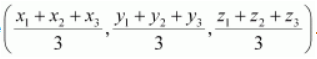.

Therefore, coordinates of the centroid of ΔPQR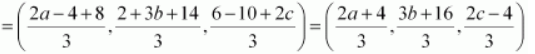It is given that origin is the centroid of ΔPQR.

Thus, the respective values ofa,b, andc are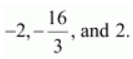Question4. Find the coordinates of a point ony-axis which are at a distance offrom the point P (3, –2, 5).

Solution :

If a point is on the y-axis, then x-coordinate and the z-coordinate of the point are zero.

Let A (0, b, 0) be the point on the y-axis at a distance of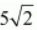from point P (3, –2, 5). Accordingly,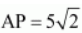Thus, the coordinates of the required points are (0, 2, 0) and (0, –6, 0).

Question5. A point R withx-coordinate 4 lies on the line segment joining the pointsP (2, –3, 4) and Q (8, 0, 10). Find the coordinates of the point R.

[Hint suppose R divides PQ in the ratio k: 1. The coordinates of the point R are given by

Solution :

The coordinates of points P and Q are given as P (2, –3, 4) and Q (8, 0, 10).

Let R divide line segment PQ in the ratio k:1.

Hence, by section formula, the coordinates of point R are given by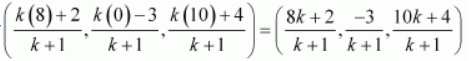It is given that the x-coordinate of point R is 4.

Therefore, the coordinates of point R areQuestion6. If A and B be the points (3, 4, 5) and (–1, 3, –7), respectively, find the equation of the set of points P such that PA2 + PB2 =k2, wherek is a constant.

Solution :
The coordinates of points A and B are given as (3, 4, 5) and (–1, 3, –7) respectively.

Let the coordinates of point P be (xyz).

On using distance formula, we obtain

Now, if PA2 + PB2 = k2, then

Thus, the required equation is<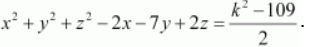Get 30% off your first purchase!

X
error: Content is protected !!
Scroll to Top